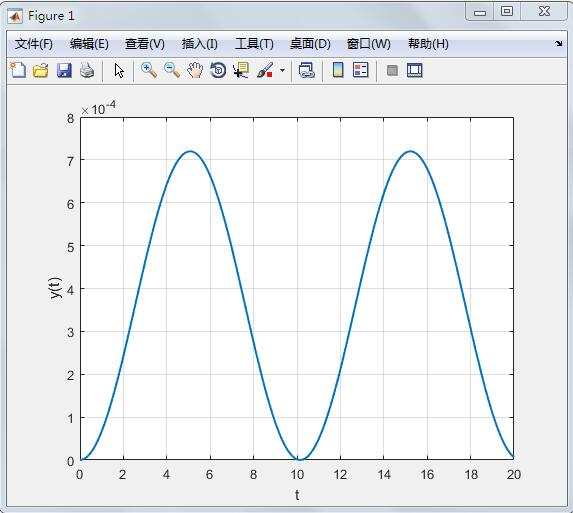# 二阶曲线方程标准形式_matlab 求解二阶微分方程并画出y与t函数曲线

x=t
y=t
z=t

x=t*20
y=t*20
z=t*10

### 已知二阶曲线上一点p,求作p点的切线

y-k=(2aj+b)(x-j) 就是切线. ps,这不是你今天上午的考试题目吧?!

### matlab用三种方法求解二阶微分方程x''+0.2x'=0.4x=0.2u(t),u(t)是单位阶跃函数,初始状态为0

x''+0.2x'-0.4x=0.2u(t), x(0)=x'(0)=0.
1.你用Laplace Transform 做吧.
L(x'')+0.2L(x')-0.4L(x)=0.2L(u(t))
(p^2)*X(p)-p*x(0)-x'(0)+0.2(p*X(p)-x(0))-0.4*X(p)=0.2/p,
(p^2+0.2*p-0.4)*X(p)=0.2/p,
X(p)=0.2/(p^3+0.2*(p^2)-0.4p)

F=0.2/(s^3+0.2*s^2-0.4*s)；
x(t)=ilaplace(F,s,t)

2.令x1=x,x2=x1'=x',把原方程转化成微分方程组：
x1'=x2
x2'=0.4*x1-0.2*x2+0.2u(t),
(x1(0),x2(0))=(0,0).

function ydot=DyDt(t,y)
if t>0
u(t)=1
else
u(t)=0
end
ydot=[y(2);0.4*y(1)-0.2*y(2)+0.2*u(t)];
tspan=[0,30];%solution interval
y0=[0;0];
[tt,yy]=ode45(@DyDt,tspan,y0)
3.用差分法吧.
h=t/N, N是你给定的正整数,一般取20吧.ti=it/N.如果t>0
x''(ti)=(x(i+1)-2x(i)+x(i-1))/(h^2)
x'(ti)=(x(i+1)-x(i-1))/2h
x(ti)=x(i)
u(ti)=u(i)=1

(x(i+1)-2x(i)+x(i-1))/(h^2)+0.2*(x(i+1)-x(i-1))/2h-0.4*x(i)=0.2

### matlab 求解二阶微分方程并画出y与t函数曲线

用matlab 求解二阶微分方程并画出y与t函数曲线，可以用dsolve函数和plot函数来实现。 　　给出的二阶微分方程存在着几个疑点：1、表达式中的z是否是笔误，还是y。如是z，其表达式是什么？2、求解二阶微分方程的数值解，必须有两个初始条件，本题只有一个。 　　下列代码中，z按y来处理，增加初始条件y'(0)=0 　　>> m=0.00267;k=0.5;b=0.000001;a=0.000004;U=90;p=-19.5216;A=0.001256; 　　>> syms y(t) 　　>> Dy=diff(y,1);D2y=diff(y,2); 　　>> y=dsolve(m*D2y+(k+1/b)*y==a/b*U-p*A,y(0)==0,Dy(0)==0); 　　>> t=0:0.1:20; 　　>> y=eval(y); 　　>> plot(t,y)### 曲线系方程是怎么推出来的? 20分

我个人的见解是，这种二次曲线系方程没有什么“推出来”之说。曲线系方程的设法可以说无限多，但我们需要找的是一个既简洁，而且又能通过变换参数得到所有符合要求的二次曲线的曲线系方程。你所说的就是一个，据我所知是没有限制的。具体怎么找，就无从得知了。 　　我在学习中也对此有很大疑惑，以上只是我自己的想法，可能不怎么好吧。 　　我还记得别的几个。以下提到的曲线均为二次曲线。方程均为标准方程 　　曲线G1，G2相交：a*G1+b*G2=0 　　l1,l2与曲线G各有两个交点，则过这四个交点的曲线系方程可令为：a*l1*l2+bG=0 　　过不共线四个点，A,B,C,D的二次曲线：a*AB*BC+b*CD*DA*=0 　　暂时难以查证，希望没错

### 圆锥曲线一般方程是什么，怎么求呢

现在新课标都教矩阵了吧，请允许我用相关知识解释一下。圆锥曲线是二次曲线，教材上的圆锥曲线方程，只是标准方程。 　　二次曲线的一般方程是：Ax^2+By^2+Cxy+Dx+Ey+F=0 　　这个方程表示什么呢？——表示所有的二次曲线，包括圆、椭圆、双曲线、抛物线、点、双直线图形和无轨迹。这些图形可以是任意平移旋转过的。 　　如果给定方程Ax^2+By^2+Cxy+Dx+Ey+F=0，要判断曲线类型，这时候直接看是不容易看出来的，就需要做一些处理。 　　（1）先考虑退化的曲线——双直线和点，当且仅当行列式Det3= 　　|A C/2 D/2| 　　|C/2 B E/2 | = 0 时, 　　|D/2 E/2 F | 　　二次曲线是退化的。这时，如果det2=AB-C^2/4=0则是椭圆退化成了一点；如果不等于0，就是直线。 　　如果是直线，先把A化成正的， 　　①平行或重合直线，由(ax+by+c)(ax+by+d)=0展开对比得，AB是同号的。 　　当D/E=√(A/B)或者是D√B=E√A，且C=2√(AB)时，两直线斜率一样，此时，若2F=D/√A或2F=E/√B，则重合，否则平行。如果要求直线，则a=√A，b=√B，c+d=D/√A=E/√B，cd=F 　　②相交直线，不符合①的双直线就是相交直线，如果A=-B，则分解因式验证其是否垂直。 　　（2）对于非退化的二次曲线，Det3≠0，这时看 　　Det2= 　　|A C/2| 　　|C/2 B | 　　即Det2=AB-C^2/4 　　Det2>0，椭圆，如果A=B则是圆；如果Det1=A+B>0（先把A化成正的）、且Det3>0，则是无轨迹的图形(不算退化)。 　　Det2<0，双曲线； 　　Det2=0，抛物线。 　　---------------------- 　　再说一下退化，对于标准形式， 　　椭圆左右各除以无穷大，就有x^2/a^2+y^2/b^2=0，就退化成了一点。 　　双曲线退化，x^2/a^2-y^2/b^2=0，退化为相交双直线，也就是她的渐近线。 　　抛物线退化，y^2=a，退化成了平行或重合的双直线。 　　三种曲线和他们的退化形式，经过旋转和平移，上文Det1、Det2、Det3的符号特征是不变的，所以可以这样判断，这三个值，称为二次曲线的不变量。

### 高等数学 微分方程 为什么一阶的求曲线，二阶是斜率，不懂，求解答，原理是什么?_?

不知道你怎么看书的，语文没学好啊。。。。所有的最终都是求曲线的方程，只不过 　　（1）第一个是已知了一阶微分（即导数），和某点的初值。几何意义就是已知了一阶微分和某点的值，要求该曲线 　　（2）第二个是已知二阶微分，在某点的值及其导数的值（导数的值即斜率呗）。几何意义就是有一条曲线，它的二阶微分是知道的，并且它过了某点，并且在该店的斜率也是已知的，要求曲线方程

### 如何由传递函数写出微分方程 求步骤

我的解答 楼主不懂的可以问我### 含有三角函数的二阶微分方程的特解怎么求

得是常系数的二阶非齐次线性方程才可以的，如图所示圈3### 急求！！关于二阶常微分方程的积分曲线怎么求！

（dy）² -2dxdy -3（dx）² =0， 　　所以(dy-3dx)(dy+dx)=0, 　　所以dy-3dx=0,或dy+dx=0, 　　积分得y-3x=c,或y+x=d.(c,d是常数).

### 高数题：x=3是曲线方程的3重根，为什么它是方程二次导数的单根？

设方程为F(x)=0，三重根为x=x0 　　则F(x)=f(x)*(x-x0)^3 f(x)是多项式 　　F'(x)=f '(x)*(x-x0)^3 + f(x)*3*(x-x0)^2 　　F''(x)=f ''(x)*(x-x0)^3 + f '(x)*3*(x-x0)^2 + f '(x)*3*(x-x0)^3 + 6*f(x)*(x-x0) 　　=(x-x0) * [ f ''(x)*(x-x0)^2 + f '(x)*3*(x-x0) + f '(x)*3*(x-x0)^2 + 6*f(x)] 　　F''(x0)=0 　　如果是重根的话，则有f(x0)=0，即f(x)=g(x)*(x-x0)， g(x)是多项式 　　于是F(x)=g(x)*(x-x0)^4 　　说明x0至少是F(x)=0的四重根，矛盾。所以是单根。 　　---------------------- 　　看具体情况吧。反正就是看一阶导数和二阶导数的情况。快速做出来也就是特殊情况比如这题，能够快速判断一阶导数和二阶导数的零点。

### 证明 ：任意平面二次曲线T都存在等矩变换F 使F(T)的方程为标准型

平面二次曲线的二次项可以用一个二次型来表示，其中二次型的矩阵A是实对称矩阵，则A必然可以对角化。也就是存在一个正交矩阵T(它就是正交变换对应的矩阵)，使得A变为对角矩阵D，从而二次曲线的方程中诸如xy,yz的交叉项消去，只剩下平方项，也就是变为标准型方程了。

### 已知曲线 ： ，若矩阵 对应的变换将曲线 变为曲线 ，求曲线 的方程.

试题分析：解决本题关键有两点，一是熟练掌握二阶矩阵左乘向量的运算，即 ，主要注意点是对应；二是利用“相关点法”求轨迹方程.根据原曲线上点与对应点的关系 ，及 ，平方相减得 ，从而解出所求轨迹方程.试题解析：解：设曲线 一点 对应于曲线 上一点 ， ， ， ， 5分 ， ， ， 曲线 的方程为 . 10分~~~~~~